# Decimal to Fraction Conversion Worksheets

#### How to Convert Between Decimals and Fractions

There are different number systems in mathematics, but there are two basic categories. These are rational and irrational numbers. Rational numbers are those that can be written in the form of fractions, and irrational numbers are the ones that one cannot write in the form of fractions. So, how do you convert rational decimal numbers into a fraction? The first step of the process is to remove the decimal. To remove the decimal, you have to move it to the point that turns the decimal number into a whole number and add zeros in the denominator equal to the number of places you shift the decimal. As you have successfully converted the number into a fraction, the final step is to bring the fraction in its simplest form.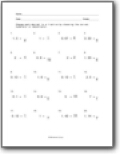###### Tenths

Change each decimal to a fraction by choosing the correct numerator or denominator. The values here are ranged to the tenths.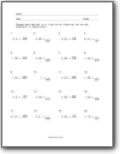###### Hundredths Place

Same skill, but these expand to the hundredths place.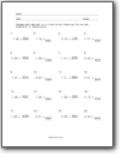###### Thousandths

We get a bit outside the lines here and step up our decimal value.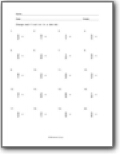###### Single Digit Fractions

Change each fraction to a decimal. All the fractional values are only single digits.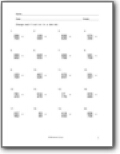###### Working with Hundredths Fractions

Convert the fractions that are written in the hundreds to decimals.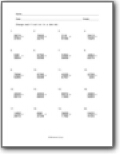###### Convert Thousandths to Decimals

You will be given a fractional value in thousandths that you need to convert to a decimal value.